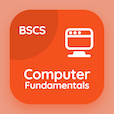Engineering Online Courses

Electromagnetic Theory Quizzes

Electromagnetic Theory Quiz PDF - Complete

# Wave Vector Quiz Questions Online p. 31

Learn Wave Vector quiz questions and answers, wave vector MCQ with answers PDF 31 to learn Electromagnetic Theory online course. Metamaterials trivia questions, Wave Vector Multiple Choice Questions (MCQ) for online college degrees. "Wave Vector Quiz" PDF Book: atoms and molecules, dielectric permittivity, boundary conditions, introduction to electromagnetic theory, wave vector test prep for job placement test.

"If k= wave vector and E is the Electric field than kxE=" MCQ PDF: ∊we, µ+wh, µwh, and ∊+we for tricky trivia questions. Study metamaterials questions and answers to improve problem solving skills to apply to colleges online.

## Quiz on Wave Vector MCQs

MCQ: If k= wave vector and E is the Electric field than kxE=

µ+wH
∊wE
µwH
∊+wE

MCQ: Electromagnetic field theory is a discipline concerned with the study of charges

at Rest
in Motion
at rest and in Motion
None

MCQ: A boundary condition which specifies the value of the normal derivative of the function is a

Neumann boundary condition
Neument boundary condition
Neumornn boundary condition
Deumann boundary condition

MCQ: Square root of the relative permitivity is referred to as

index of reflection
index of refraction
index of absorption

MCQ: Revolving electrons present in various shells and surround the nucleus, exert a force on nucleus which is

force of repulsion
gravitational force
acceleration force
force of attraction

### More Quizzes from Electromagnetic Theory Course